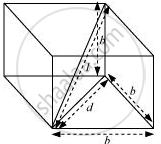Share

# A Cube of Side B Has a Charge Q At Each of Its Vertices. Determine the Potential and Electric Field Due to this Charge Array at the Centre of the Cube. - CBSE (Science) Class 12 - Physics

ConceptPotential Energy of a System of Charges

#### Question

A cube of side has a charge at each of its vertices. Determine the potential and electric field due to this charge array at the centre of the cube.

#### Solution

Length of the side of a cube = b

Charge at each of its vertices = q

A cube of side is shown in the following figure.d = Diagonal of one of the six faces of the cube

d^2=sqrt(b^2+b^2)=sqrt(2b^2)

d=bsqrt2

l = Length of the diagonal of the cube

l^2=sqrt(d^2+b^2)

=sqrt((sqrt(2)b)^2)+b^2=sqrt(2b^2+b^2)=sqrt(3b^2)

l=bsqrt3

r=1/2=(bsqrt3)/2 is the distance between the center of the cube and one of the eight vertices

The electric potential (V) at the centre of the cube is due to the presence of eight charges at the vertices.

V=(8q)/(4piin_0)

=(8q)/(4piin_0(bsqrt3/2))

=(4)q/(sqrt3 pi in_0 b).

Therefore, the potential at the centre of the cube is (4)q/(sqrt3 pi in_0 b).

The electric field at the centre of the cube, due to the eight charges, gets cancelled. This is because the charges are distributed symmetrically with respect to the centre of the cube. Hence, the electric field is zero at the centre.

Is there an error in this question or solution?
Solution A Cube of Side B Has a Charge Q At Each of Its Vertices. Determine the Potential and Electric Field Due to this Charge Array at the Centre of the Cube. Concept: Potential Energy of a System of Charges.
S